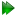Stereochemistry - the sample script

The following script was used for the analysis in Sun et al. (2011). It is used to complete the first step of the analysis, the determination of relative configuration, and for the generation of ensembles for the second step of the analysis. The file is located at sample_scripts/n_state_model/stereochem_analysis.py. The contents of the script are:

"""Script for the determination of relative stereochemistry.

The analysis is preformed by using multiple ensembles of structures, randomly sampled from a given set of structures.  The discrimination is performed by comparing the sets of ensembles using NOE violations and RDC Q factors.

This script is split into multiple stages:

1.  The random sampling of the snapshots to generate the N ensembles (NUM_ENS, usually 10,000 to 100,000) of M members (NUM_MODELS, usually ~10).  The original snapshot files are expected to be named the SNAPSHOT_DIR + CONFIG + a number from SNAPSHOT_MIN to SNAPSHOT_MAX + ".pdb", e.g. "snapshots/R647.pdb".  The ensembles will be placed into the "ensembles" directory.

2.  The NOE violation analysis.

3.  The superimposition of ensembles.  For each ensemble, Molmol is used for superimposition using the fit to first algorithm.  The superimposed ensembles will be placed into the "ensembles_superimposed" directory.  This stage is not necessary for the NOE analysis.

4.  The RDC Q factor analysis.

5.  Generation of Grace graphs.

6.  Final ordering of ensembles using the combined RDC and NOE Q factors, whereby the NOE Q factor is defined as::

Q^2 = U / sum(NOE_i^2),

where U is the quadratic flat bottom well potential - the NOE violation in Angstrom^2. The denominator is the sum of all squared NOEs - this must be given as the value of NOE_NORM.  The combined Q is given by::

Q_total^2 = Q_NOE^2 + Q_RDC^2.
"""

# relax module imports.
from auto_analyses.stereochem_analysis import Stereochem_analysis

# Stage of analysis (see the docstring above for the options).
STAGE = 1

# Number of ensembles.
NUM_ENS = 100000

# Ensemble size.
NUM_MODELS = 10

# Configurations.
CONFIGS = ["R", "S"]

# Snapshot directories (corresponding to CONFIGS).
SNAPSHOT_DIR = ["snapshots", "snapshots"]

# Min and max number of the snapshots (corresponding to CONFIGS).
SNAPSHOT_MIN = [0, 0]
SNAPSHOT_MAX = [76, 71]

# Pseudo-atoms.
PSEUDO = [
["Q7",  ["@H16", "@H17", "@H18"]],
["Q9",  ["@H20", "@H21", "@H22"]],
["Q10", ["@H23", "@H24", "@H25"]]
]

# NOE info.
NOE_FILE = "noes"
NOE_NORM = 50 * 4**2    # The NOE normalisation factor (sum of all NOEs squared).

# RDC file info.
RDC_NAME = "PAN"
RDC_FILE = "pan_rdcs"
RDC_SPIN_ID1_COL = 1
RDC_SPIN_ID2_COL = 2
RDC_DATA_COL = 2
RDC_ERROR_COL = None

# Bond length.
BOND_LENGTH = 1.117 * 1e-10

# Log file output (only for certain stages).
LOG = True

# Number of buckets for the distribution plots.
BUCKET_NUM = 200

# Distribution plot limits.
LOWER_LIM_NOE = 0.0
UPPER_LIM_NOE = 600.0
LOWER_LIM_RDC = 0.0
UPPER_LIM_RDC = 1.0

# Set up and code execution.
analysis = Stereochem_analysis(
stage=STAGE,
num_ens=NUM_ENS,
num_models=NUM_MODELS,
configs=CONFIGS,
snapshot_dir=SNAPSHOT_DIR,
snapshot_min=SNAPSHOT_MIN,
snapshot_max=SNAPSHOT_MAX,
pseudo=PSEUDO,
noe_file=NOE_FILE,
noe_norm=NOE_NORM,
rdc_name=RDC_NAME,
rdc_file=RDC_FILE,
rdc_spin_id1_col=RDC_SPIN_ID1_COL,
rdc_spin_id2_col=RDC_SPIN_ID2_COL,
rdc_data_col=RDC_DATA_COL,
rdc_error_col=RDC_ERROR_COL,
bond_length=BOND_LENGTH,
log=LOG,
bucket_num=BUCKET_NUM,
lower_lim_noe=LOWER_LIM_NOE,
upper_lim_noe=UPPER_LIM_NOE,
lower_lim_rdc=LOWER_LIM_RDC,
upper_lim_rdc=UPPER_LIM_RDC
)
analysis.run()

In contrast to all of the other auto-analyses, here you do not set up your own data pipe containing all of the relevant data that is then passed into the auto-analysis. This may change in the future to allow for more flexibility in how you load structures, load the RDC and NOE base data, set up pseudo-atoms and bond lengths for the RDC, etc.

Note that you need to execute this script 6 times, changing the STAGE variable to match. These stages are fully documented at the start of the script.

Due to the original analysis being performed prior to the addition of the structure.superimpose user function to relax, you will see that the auto-analysis performs superimposition of each ensemble using the external software Molmol. If you wish to perform this analysis without using Molmol, please contact the relax users mailing list “nmr-relax-users at lists.sourceforge.net” (see Section 3.3.2 on page). It would be rather straightforward for the relax developers to replace the complicated Molmol superimposition code with a single call to the structure.superimpose user function.

The relax user manual (PDF), created 2020-08-26.